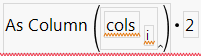Our World Statistics Day conversations have been a great reminder of how much statistics can inform our lives. Do you have an example of how statistics has made a difference in your life? Share your story with the Community!
Choose Language Hide Translation Bar

## Insert one expression into another using Eval Insert, Eval Expr, Parse, and Substitute

Problem

You need to insert the value of a variable into an expression without evaluating the whole code.  Examples include:

1. Writing column formulas using a script
2. Writing scripts using another script

Below is a script to transform the selected columns in a table. This does NOT work, because the expression 'cols[i]' is saved in the column formula instead of the column name.

``````//Open A Table and select a few columns
dt = Open( "\$Sample_data/iris.jmp" );
cols = {Sepal length, Petal length};

//This code does not work
For( i = 1, i <= N Items(cols), i++,
dt << New Column(
"2 * " || Char( cols[i] ),
Numeric,
"Continuous",
Formula( As Column( cols[i] ) * 2)
)
);``````

New columns are created with the correct name but this formula:Solution

There are two general strategies to solve this: building the expression while evaluating part of it before the rest, and building the expression as a string and then evaluating it. Note that the variable being inserted can actually be an expression of any length.

Expressions
These methods involve writing an expression and evaluating one part of it before the rest. It can be tricky to find the right combination of Eval, Eval Expr, and Expr, but you can see syntax highlighting and use the script editor to make sure parenthesis are matched.

Eval, Eval Expr
Write the expression and wrap any parts that should be evaluated immediately with Expr():
``````//Eval Expr
For( i = 1, i <= N Items(cols), i++,
Eval( Eval Expr(
dt << New Column(
"2 * " || Char( cols[i] ),
Numeric,
"Continuous",
Formula( As Column( Expr( Char( cols[i] ) ) ) * 2)
)
) )
);``````
Substitute
Write the expression and replace part of it with evaluated code:
``````//Substitute
For( i = 1, i <= N Items(cols), i++,
Eval( Substitute(
Expr( dt << New Column(
"2 * " || cn,
Numeric,
"Continuous",
Formula( As Column( cn ) * 2)
) ),
Expr( cn ),
Char( cols[i] )
) )
);``````

String Methods
These methods involve writing the expression as a string, using Parse() to convert it into an expression and then Eval() to actually evaluate it. Downsides of these methods include not seeing any syntax highlighting, needing to handle double-quotes using either \!" or \[ ... ]\, and being generally hard to troubleshoot or modify in the future, especially for long expressions.

Eval Insert
Write the expression as a string with 'in-line' code that is evaluated and placed inside the string, then parse and evalute it:
``````//Eval Insert
For( i = 1, i <= N Items(cols), i++,
Eval( Parse( Eval Insert(
"\[dt << New Column(
"2 * ^Char( cols[i] )^",
Numeric,
"Continuous",
Formula( As Column( "^Char( cols[i] )^" ) * 2)
)]\"
) ) );
);``````
Substitute
Write the expression in a string with a placeholder that is replaced with the evaluated expression. Note this is the same function as above:
``````//Substitute
For( i = 1, i <= N Items(cols), i++,
Eval( Parse( Substitute(
"\[dt << New Column(
"2 * ColumnName",
Numeric,
"Continuous",
Formula( As Column( "ColumnName" ) * 2)
)]\",
"ColumnName",
Char( cols[i] )
) ) );
);``````
Concatenate
The most basic form, write the expression using '||'.  Note you could use use \[ ... ]\ instead of \!":
``````//Concatenate
For( i = 1, i <= N Items(cols), i++,
Eval( Parse(
"dt << New Column(
\!"2 * " || Char( cols[i] ) || "\!",
Numeric,
\!"Continuous\!",
Formula( As Column( \!"" || Char( cols[i] ) || "\!" ) * 2)
)"
) );
);``````

The 'Expression Handling Functions', section of the Scripting Guide includes these and other methods to accomplish the same thing.  This blog post by @joseph_morgan has addition examples and insights into the same topic.

Thank you @Justin_Chilton for feedback on this post.